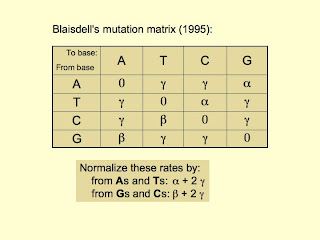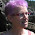## Field of Science

• 1 year ago in PLEKTIX
• 1 year ago in History of Geology
• 1 year ago in Angry by Choice
• 2 years ago in Chinleana
• 3 years ago in Field Notes
• 4 years ago in Moss Plants and More
• 5 years ago in Rule of 6ix
• 5 years ago in Games with Words
• 6 years ago in Protein Evolution and Other Musings
• 6 years ago in The View from a Microbiologist
• 7 years ago in Memoirs of a Defective Brain
• 9 years ago in Sex, Genes & Evolution
• 10 years ago in Life of a Lab Rat
• 10 years ago in Disease Prone
• in The Biology Files

### Controlling mutagenesis

I've made some more progress on the problem of how to score USSs in simulated DNA fragments, and written it up in our work-in-progress document of how our model works. But here I want to get back to thinking of how mutagenesis can be set up to allow control of both the base composition and the ratio of transition mutations to transversion mutations. The problem was introduced in a previous post.

I have a printout of the analysis done for us by my biomathematician colleague. I'll try to summarize here what I think it says, and then I'll ask her to look at this post and tell me if I've got it wrong.Before our program does any mutagenesis it will need to first calculate the values of three parameters, alpha, beta and gamma. (spelled out because Blogger doesn't do Greek letters.) These parameters specify the rates at which the specific bases mutate to each of the other three bases, as indicated in the table ("Blaisdell's 1985 mutation matrix"). In our model we will normalize these by dividing by their sums, as indicated below the table, so we can use them as probabilities.The values these parameters will take depend on the three parameters we give to the model; these are described in the blue box. The formulas in the green box were derived by our colleague - we will write Perl code that uses these to calculate alpha, beta and gamma from G, µ and R. The program will then put these values into the table. Then, at each mutation step, the program will determine whether the mutating base is an A, T, C or G, and look up the appropriate probabilities of bases it can mutate to.

If we were to begin the simulation with a genome that did not have the desired base composition, it would also not initially have the desired ratio of transitions to transversions. If no opposing forces were acting, the base composition and ratio would equilibrate at the desired values. This should not be an issue for us because our genomes will be created with the desired base compositions.

1.Hi Rosie,

This looks fine to me with just some minor adjustments.

There are two types of matrices that are represented in the literature, depending on whether time is measured in discrete steps (like a year) or in continuous time.

In the first case (discrete time steps), you should have elements like 1-2gamma-alpha (first row, first column) along the diagonal in the matrix. For example, the probability that an A remains an A over one year would be 1-2gamma-alpha.

In the second case (continuous time), the matrix represents a rate per unit time of changing from state i to state j. In this case, the element in the first row and first column would be -2gamma-alpha. Then, the rate of change of the proportion of As per unit time could be written as a differential equation:

dA/dt = (-2gamma-alpha)*A+(delta)*T+(delta)*C+(beta)*G

The most likely type of simulation that you would want to run would run time in particular time units, with each loop corresponding to one time step (e.g., a cell replication, an hour, a year, or whatever). The first matrix would then tell you the fraction of As that stay the same (1-2gamma-alpha), the fraction that mutate to C (gamma), etc, within each loop.

Note that you don't have to normalize these rates by dividing by the sum. You would do that only if you wanted to say that the probability that A mutates to something is 2gamma+alpha, after which point you could say that the fraction of the mutations going to C is gamma/(2gamma+alpha), the fraction going to T is gamma/(2gamma+alpha), and the fraction going to G is alpha/(2gamma+alpha). But this normalization isn't necessary if you just run your simulations using the matrix to give the probability of moving from state i to j in a loop.

Let me know if it converges to the right equilibrium!

Cheers,
Sally

2.Because our genomes are very big and only a small fraction of bases mutate in any one cycle, the program won't scan every base and use the matrix to decide whether it stays the same or mutates. Instead it will first decide how many bases will mutate, randomly choose which positions in the genome these will be, and then use the matrix to decide which base each mutation changes to.

That's why the 'no change' cells of the matrix have values of 0. I'll let you know if the calculations don't give the desired base compositions.

Markup Key:
- <b>bold</b> = bold
- <i>italic</i> = italic
- <a href="http://www.fieldofscience.com/">FoS</a> = FoS